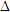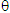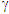$$\require{cancel}$$

# Resources: Formulae, Constants, and Conversions

Formulae

Relationship

Initial Chapter= c/ f  wavelength, frequency, and speed Chapter 2
E = hf energy and frequency
Ephoton =Eelectron energy of photon emitted/absorbed by atom
En = -13.6/n2 energy levels of atom
T = 2.9e-3/temperature and peak wavelength
F =T4 brightness and temperature
A =r2 area and radius Chapter 3= 1.22/D resolution
v = d/t speed, distance, and time Chapter 4
z =/redshift
v = cz velocity and redshift
t = E/L stellar lifetime Chapter 5
E = mc2 energy and mass
t ~ m-2 stellar lifetime and mass
L ~ m3 stellar mass and luminosity
d = 1/a  parallax angle and distance Chapter 6
d = S/small angle formula
F= L/4d2 inverse square law
F = ma Newton's second law Chapter 7
Fg = mg Weight and mass
ac = v2/r Centripetal acceleration
Fg = Gm1m2/r2 Newton's law of gravity
PE = mgh Potential energy (non-relativistic)
KE = ½ mv2 Kinetic energy (non-relativistic)
Efinal = Einitial Conservation of energy
vescape = (2GM/R)½ Escape velocity
v ∝ r Rotation of rigid disk Chapter 8
v ∝ 1/r Rotation of water around drain
v ∝ constant Rotation of cars on roundabout
v ∝ 1/r½ Keplerian rotation (planets)
v2 = GM/r Relationship of enclosed mass to velocity and distance
M = ρV Mass, density, and volume= 1/√(1-v2/c2) gamma factor Chapter 9t’ =t time dilation
L’ = L/length contraction
d2 =x2 +y2 Pythagorean Theorem
s2 =x2 – c(t)2 spacetime interval
E = mc2 mass and rest energy
E =E0 total energy and rest energy
\begin{equation} g = \frac{GM}{R^2} \end{equation} Surface gravity Chapter 10
\begin{equation} d=v_0t+\frac{1}{2}at^2 \end{equation} Distance and acceleration
\begin{equation} v=at \end{equation} Velocity and acceleration
\begin{equation} t=\frac{t_0}{\left(1-\frac{gH}{c^2}\right)} \end{equation} Time dilation (weak field approximation)
\begin{equation} f=f_0\left(1-\frac{gH}{c^2}\right) \end{equation} Gravitational redshift (weak field approximation, frequency, photon traveling upward)
\begin{equation} \lambda=\frac{\lambda_0}{\left(1-\frac{gH}{c^2}\right)} \end{equation} Gravitational redshift (weak field approximation, wavelength)
\begin{equation} f=f_0{\sqrt{1-\frac{2GM}{Rc^2}}} \end{equation} Gravitational redshift (full expression, frequency)
\begin{equation} \lambda=\frac{\lambda_0}{\sqrt{1-\frac{2GM}{Rc^2}}} \end{equation} Gravitational redshift (full expression, wavelength)
\begin{equation} d^2=\left(\Delta x\right)^2 + \left(\Delta y\right)^2 \end{equation} Pythagorean theorem
\begin{equation} d^2=\left(R\Delta\theta\right)^2+\cos^2\theta\left(R\Delta\alpha\right)^2 \end{equation} Distance on a sphere
\begin{equation} d^2=\left(\Delta x\right)^2 + \left(\Delta y\right)^2 +\left(\Delta z\right)^2 \end{equation} Pythagorean Theorem in 3-D
\begin{equation} s^2=\left(\Delta x\right)^2+\left(\Delta y\right)^2 + \left(\Delta z\right)^2-\left(c\Delta t\right)^2 \end{equation} Spacetime interval in flat space
\begin{equation} s^2=\left(1-\frac{2GM}{rc^2}\right)^{-1}\left[\left(\Delta x\right)^2+\left(\Delta y\right)^2 + \left(\Delta z\right)^2\right]-\left(1-\frac{2GM}{rc^2}\right)\left(c\Delta t\right)^2 \end{equation} Spacetime interval in spherically curved space
\begin{equation} \theta = \frac{2GM}{bc^2} \end{equation} Angle of deflection of light
\begin{equation} P_{gw}=\frac{2}{5}\left(\frac{GM}{Rc^2}\right)^5\left(\frac{m}{M}\right)^2\left(\frac{c^5}{G}\right) \end{equation} Power emitted by gravitational waves
\begin{equation} {\rm\bf{G}}={8\pi} G ~{\rm\bf{T}}/c^4 \end{equation} Einstein equation
\begin{equation} R_S = \frac{2GM}{c^2} \end{equation} Schwarzschild radius Chapter 11
\begin{equation} s^2=\left(1-\frac{R_{S}}d\right)^{-1} (\Delta d)^2 - \left( 1-\frac{R_{S}}{d}\right) (c\Delta t)^2 \end{equation} Spacetime interval in a spherically symmetric space (Schwarzschild interval)
\begin{equation} T_{bh}=\frac{1.23 \times 10^{23}}{M} \end{equation} Temperature of a black hole
\begin{equation} \Delta E \Delta t \geq \frac{h}{4\pi} \end{equation} Uncertainty principle
\begin{equation} L=\frac{3.56\times10^{32}}{M^2} \end{equation} Luminosity of a blackhole
\begin{equation} t \approx 2.5\times 10^{-16} M^3 \end{equation} Evaporation time
\begin{equation} \frac{\Delta m}{\Delta t}=-\frac{2L}{c^2} \end{equation} Accretion rate
\begin{equation} L_{edd} = 6.3 M_{BH} \end{equation} Eddington luminosity
\begin{equation} \alpha = \frac{4GM}{bc^2} \end{equation} Deflection angle (full) Chapter 12
\begin{equation} \theta^2-x\theta-\theta_E^2=0 \end{equation} Lens equation
\begin{equation} m = \frac{1}{\left[1-\left(\frac{\theta_E}{\theta}\right)^4\right]} \end{equation} Magnification for a point-mass lens
\begin{equation} v = H_0 d \end{equation} Hubble law Chapter 13
\begin{equation} v = cz \end{equation} Cosmological redshift
\begin{equation} d_{\rm physical}(t) = d_{\rm comoving}(t) S(t) \end{equation} Comoving coordinates
\begin{equation} 1+z=\frac{S(t_{\rm observed})}{S(t_{\rm emitted})} \end{equation} Ratio of scale factors
\begin{equation} t = \frac{1}{H_0} \end{equation} Hubble time (age)
\begin{equation} H^2 - \frac{8 \pi G \rho}{3} = - \frac{k c^2}{S^2} \end{equation} Friedman equation
d = cz/H0 distance and redshift Chapter 14
Te / To = 1 + z = So / Se Temperature, redshift, and scale factor Chapter 15
E ~ kT energy and temperature Chapter 16
T ~ mc2/k temperature of Universe and mass of particle in reaction

Constants

Name

c = 3 x 108 m/s = 3 x 105 km/s speed of light
h = 6.63 x 10-34 J s = 4.136e-15 eV s Planck’s constant
G = 6.67 x 10-11 N m2/kg2 Universal gravitational constant
kB = 1.38 × 10-23 J/K Boltzmann's constant
tplanck ~ 10-43 s Planck time
tplanck ~ 4 × 10-35 m Planck length
me = 9.1 × 10-31 kg mass of an electron

Conversions

Units

1 km = 1000 m km and meters
1 km = 0.6 mi km and miles
1 AU = 1.5 x 1011 m = 1.5 x 10km AU and meters and km
1 ly = 9.5 x 1015 m = 9.5 x 1012 km light-years and meters and km
1 ly = 6.3 x 104 AU light-years and AU
1 eV = 1.6 x 10-19 J eV and joules
1 degree = 60 arcmin degrees and arcminutes
1 arcmin = 60 arcsec arcminutes and arcseconds
1 angstrom (Å) = 1 x 10-10 meters angstroms and meters
1 pc = 3.26 ly parsecs and light-years
1 N = 0.2248 pounds newtons and pounds
1 kpc = 3.086 x 1019 m kiloparsecs and meters
1 solar mass = 2 × 1030 kg solar masses and kg
1 Mpc = 3.09 x 1022 m megaparsecs and meters
1 Mpc = 3.09 × 1019 km megaparsecs and km

Units (abbreviation)

Type of quantity

meters (m) length (SI)
kilograms (kg) mass (SI)
second (s) time (SI)
meters per second (m/s) speed (SI)
kelvin (K) temperature (SI)
miles (mi) length
astronomical unit (AU) length
year (yr) time
light-year (ly) length
light-minutes length
light-seconds length
g/cm3 density
solar masses mass
hertz (Hz) = cycles/s = 1/s = s-1 frequency (SI)
joules (J) energy (SI)
electron volts (eV) energy
watts (W) = J/s power
degrees angle
arcmin angle
arcsec angle
angstrom (Å) length
parsec (pc) length
m/s2 acceleration (SI)
newton (N) = kg m/s2 force (SI)
joules (J) = N m energy (SI)
μK micro Kelvin = 10-6 K temperature

Prefix

Meaning (in USA)

Exponent

Symbol

Tera trillion 1012 T
Giga billion 109 G
Mega million 106 M
kilo thousand 103 k
centi one-hundredth 10-2 c
milli one-thousandth 10-3 m
micro one-millionth 10-6 μ
nano one-billionth 10-9 n
pico one-trillionth 10-12 p